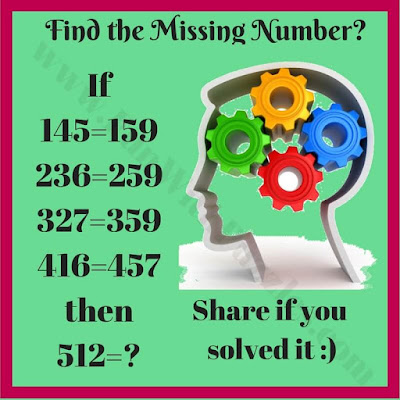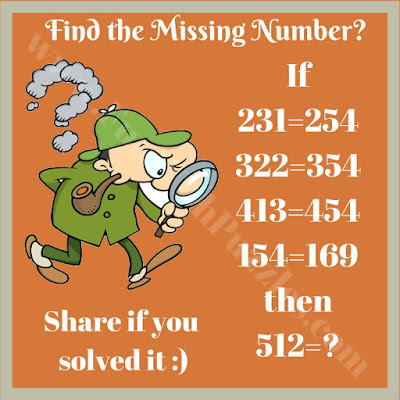Mathematical Reasoning Puzzles are those in which both Logical reasoning as well as Mathematical calculations are required to solve the puzzles. These kinds of puzzles include Circle Puzzles, Sequence Puzzles, Odd One Out Puzzles, Matchstick Puzzles and Crack the Code Puzzles etc. In all these puzzle types one has to both think logically as well as mathematically. There is another type of Mathematical Reasoning Puzzles in which some equations are given and one has to logically deduce the pattern to find which number will replace the question mark. Today's Math reasoning puzzles fall in this category.
In all of these five puzzles, some mathematical equations are given. These equations may be incorrect mathematically but if we apply logical reasoning in these equations, then these equations follow a certain pattern. One has to find this logical pattern and solve the last equations to find which number will replace the question mark.
Most of these puzzles are of equal difficulty level. However, each person may find some puzzle tough or easy depending upon their abilities. Answers to these Mathematical reasoning puzzles will be provided later. Please do post your solutions with the explanation in the comment section of this post.1. Can you crack this riddle?3. Can you solve this brain teaser?4. Can you solve this math logic picture puzzle?5. Which number replaces the question mark?

#### 1 comment:

Anonymous said...